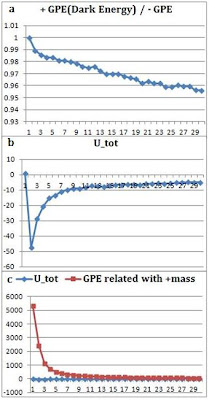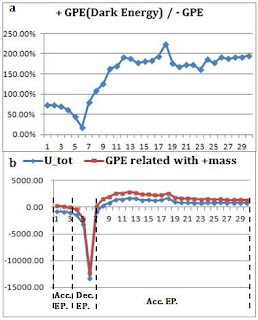I had a new computer simulation.

We set up each model from the birth of universe to the present, and calculated GPE using computer simulation in each level.

As a result, we could verify that “pair creation model of negative mass and positive mass” explains inflation of the early universe and decelerating expansion, and present accelerating expansion in time series.

This simulation is showing incredible results.
It not only explains the total energy of the universe, flatness, and the essence (Total zero energy, pair creation of negative energy and positive energy) of the process of birth of the universe, but it explains inflation, decelerating expansion in the early stage, accelerating expansion in the late stage, and dark matter through the only term, negative energy. Moreover, this negative energy is one that is essentially required by the law of energy conservation.

Simulation 1. Dark energy - Accelerating expansion of distant galaxy due to negative mass
Simulation 2. Inflation, decelerating expansion and accelerating expansion with pair creation of negative mass and positive mass
http://www.youtube.com/watch?v=SRUqQM2FfNU 3. Paper: The change of Gravitational Potential Energy and Dark Energy in the Zero Energy Universe.
http://vixra.org/abs/1110.0019

A. Birth of the universe from zero energy state
1) computer simulationFig14. m+=+1 (1,000ea), -m-=-1 (1,000ea),
U++ = -5190.4707907,
U-- = -5308.0373689,
U-+= 10499.2712222,
U_tot = 0.7630625

Total rest mass energy is zero. Total gravitational potential energy (U_tot) is +0.763.

|U_tot/U -+| = 0.0000726, so U_tot is almost zero.

We could not make GPE 0 for there were too many particles. Therefore, we simulated dividing the value of U_tot (total GPE) into two parts which are when it is little bit bigger than 0 (+0.76306) and when smaller than 0 (-0.53277), and we could gain almost similar results. (Attached in paper )

2) Accelerating expansion of the universe (inflation)

It can be confirmed that even though the total energy starts with 0, the universe expands and positive masses combine one another due to attractive interaction among themselves, while negative masses cannot form massive mass structure because of repulsive interaction.

The pair creation model of negative mass and positive mass explains “energy conservation” in times of the birth of the universe and “expansion after the birth” naturally, and it does not need institution of new mechanism or field like inflaton or inflation itself, and it explains this effect with only gravity.

3) Change of GPEFigure15-a. The ratio of negative GPE to positive GPE of the early universe. We can confirm that as the universe expands, (+GPE/-GPE) ratio decreases, and U_tot gets to be negative value. Figure15-b. Total GPE of the early universe. Figure15-c. U_tot of the early universe and GPE related with positive mass. It looks almost like a straight line for the size of U_tot is relatively small.

The graph above is that the change of GPE related with positive mass and U_tot drawn through graph.

As we have observed activities of only positive masses, “GPE related with positive mass ((U-+) + (U++))” has a significant meaning.

a) Nevertheless the value of U_tot changes from 0 to negative value, the universe expands for GPE related with positive mass has + value.

b) Note that nevertheless the total energy is 0, GPE related with positive mass has very big positive value, and this value approaches to 0 very rapidly. This explains the dramatic expansion like the early universe inflation and the finish of this inflation mechanism.

c) The thing we can notice by this and the next simulation is that if time goes bit more, U_tot and GPE related with positive mass both have negative values, and the universe is converted to the decelerating expansion stage.

d) In order to explain the flatness of the universe, typical researchers assume the inflation mechanism and explain it using this. But Zero Energy Universe does not need institution of new field for it guarantees flatness itself, and additionally, the simulation above means that the accelerating expansion of the early universe can be explained with gravity without instituting new field.

4) Change of GPE related with positive mass and U_tot in three initial valueFig16-a. Total rest mass energy=0, U_tot(initial value)= +51.79 / +0.76 / -0.53. Time scale=0.5, Step scale(+51.79,-0.53)=200, Step scale(+0.76)=250, Fig16-b. Change of GPE related with positive mass in three cases

GPE related with positive mass has very big positive value, and this value approaches to 0 very rapidly.

B. GPE among distant galaxies and accelerating expansion
1) When positive mass is spread through relatively large areaFig17.Distant galaxy – The structure that negative mass surrounds galaxy composed of positive mass.
m+ = (1 X 500) + (1 X 500) = + 1000, center1(-1000,0,0), center2(+1000,0,0), R=150,
-m- = (-1 X 500) +(-1 X 500) = - 1000, negative mass distribution :
- 250 ≤ (x ±1000),y,z ≤+250. We simulated having it has unit mass of positive mass and negative mass.

After the birth of the universe, positive masses bind together by attractive interaction. Meanwhile, negative masses are being almost uniformly distributed because of repulsive interaction. Negative masses are gravitational bounded to massive positive masses (Galaxy or Galaxy cluster) for massive positive mass has attractive effect on negative mass.Figure18-a.The ratio of +GPE to –GPE of distant galaxy. Figure18-b. U_tot and GPE related with positive mass on distant galaxy. Note that GPE value related with positive mass changes from positive value to negative value, and to positive value again. This represents acceleration expansion --> deceleration expansion --> acceleration expansion of the universe.

a) The ratio of +GPE to –GPE of distant galaxy

i) Early status is that positive GPE is smaller than negative GPE, and the U_tot has negative value. This negative GPE status results from gravitational binding of positive masses.

ii) As time goes by, binding of positive mass increases due to attractive interaction, and the absolute value of negative gravity potential reaches maximum.

iii) The absolute value of negative GPE decreases due to positive mass has a gravitational binding and negative mass does gravitational contraction and U_tot is converted to positive value as a result. Center of gravitational contraction of negative masses is galaxy or galaxy cluster.

iv) U_tot and GPE related with positive mass both are converted to positive value. Therefore, the universe gets to an era of accelerating expansion again.

v) On the simulation above, we can confirm that +GPE increases 200% the value of –GPE, and for we deduce the universal components through GPE, we will guess that repulsive dark energy increases 200% the value of attractive mass energy (matter + dark matter, as a general deduction).

b) U_tot and GPE related with positive mass on distant galaxy
i) In the early universe, GPE related with positive mass had very big + value, but this value gets smaller as positive masses binds together and comprise of galaxy structure. On the simulation above, it still has positive value, and so it is in the status of accelerating expansion.

ii) We can notice that U_tot and GPE related with positive mass both are converted to negative value by gravitational binding of positive masses. Therefore, the universe gets to an era of decelerating expansion.

iii) GPE related with positive mass is converted to positive value due to negative mass does gravitational contraction around massive positive mass(Galaxy or Galaxy Cluster). Therefore, the universe gets to an era of accelerating expansion again.

iv) The decelerating expansion and accelerating expansion is naturally explained through “pair creation model of negative mass and positive mass”, and the conversion from accelerating expansion to decelerating expansion and from decelerating expansion to accelerating expansion is explained in sequence.

v) The conversion from negative value to positive value shall be done more smoothly than the graph above for there exist thousands of billions of galaxies in our universe.Figure19-a.The change of distance and relative speed among distant galaxies. Figure19-b. GPE related with positive mass and negative mass.

c) The change of distance and relative speed among distant galaxies
Massive positive mass is given a birth from the 8th stage due to gravitational contraction. We calculated distance between the two massive positive masses (corresponding to the galaxy or galaxy cluster) and relative speeds of the two from then.

We can notice that there exits positive acceleration, and it corresponds to accelerating expansion.

d) GPE related with positive mass and negative mass
Positive mass and negative mass have different GPE value each other, therefore their movements are different each other.
Paper: The change of Gravitational Potential Energy and Dark Energy in the Zero Energy Universe.
http://vixra.org/abs/1110.0019